# Sequential Logic Circuits

Tom Kelliher, CS26

Oct. 10, 1996

• Study: A.6--A.10.

• Glance at: A.11--A.12.

# Exercises

To summarize combinational design, we'll do one or two of the following.

1. Design and implement a 2-1 multiplexer, using a K-map for minimization of the output equation. Here's the truth table: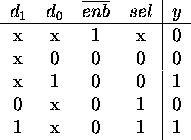A multiplexer works like a switch. One way of drawing them is: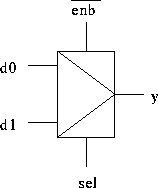2. Design and implement a circuit to take a BCD-encoded digit and drive a seven-segment display (used in watches and calculators). Use a K-map to minimize each of the seven output equations. Take advantage of don't cares. Here is the labeling for the display: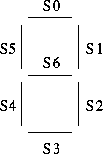3. Design and implement a circuit to take a BCD-encoded digit and increment it by one (nine should be ``incremented'' to zero). Use a K-map to minimize each of the four output equations. Take advantage of don't cares.

# Flip-Flops

1. Sequential circuits.

2. Combinational circuits have no memory.

3. Memory provided by feedback --- output becomes input in an ``earlier'' part of the circuit.

4. Clock signal --- a special control signal used to orchestrate state changes within the circuit. Types of clocked circuits:
1. Level sensitive --- state changes may occur when the clock signal is at a particular level (0 or 1).

2. Master-Slave --- The use of two clock signals with pairs of level-sensitive flip-flops to prevent ``double-clocking.'' Suffers from ``ones-catching.''

3. Edge-triggered --- state changes may occur only on a clock transition (edge).

5. State --- The values stored by sequential circuit elements (flip-flops, latches). The value of a particular signal.

Types of flip-flops:

1. R-S. Unclocked R-S: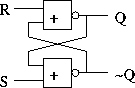Truth table: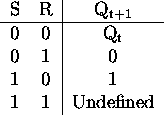Inputs always sampled --- state changes at any time.

Timing diagram?

2. J-K. Clocked (level-sensitive) J-K: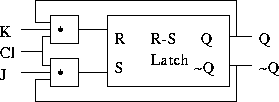Truth table: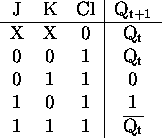Timing diagram?

Important timing nomenclature:

1. Propagation delay.

2. Set-up time.

3. Hold time.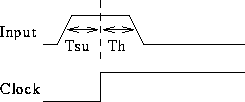Master-slave J-K: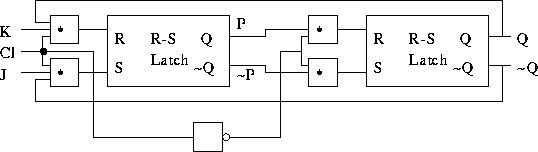Timing diagram?

3. D. Negative edge-triggered D flip-flop: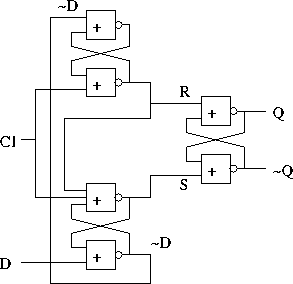Truth table: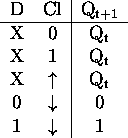How does it work? Consider:

• Clock high.

• Falling edge (inputs to 2nd stage latched).

• Clock low.

• Rising edge.

# Multiplexers and Decoders

1. Multiplexer ---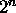-to-1 switch: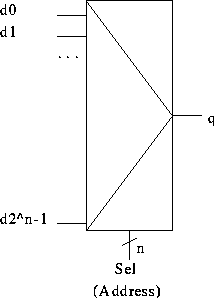``Tiling'' multiple multiplexers to switch words.

2. Decoder --- 1-of-driver: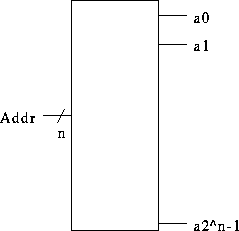# Registers

1. Tiling D flip-flops.

# Tying It All Together --- A Register File

The MIPS statement

```            add \$t0, \$t1, \$t2
```
must read two operands and write one in a single cycle.

Need a three-port register file.

General block diagram of register file: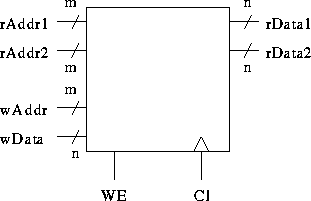Detailed block diagram: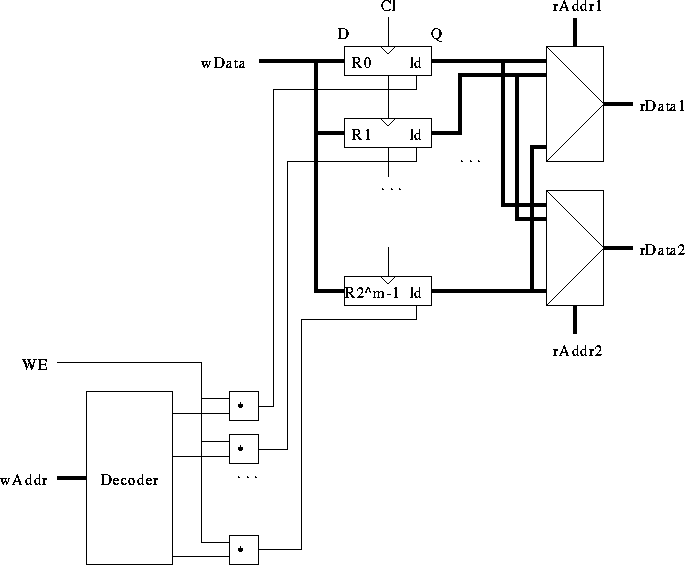Thomas P. Kelliher
Wed Oct 9 15:12:30 EDT 1996
Tom Kelliher